Hu, Y., and Xue, B. (2013). "Analysis of nondestructive testing for mechanical properties of LVL at different relative humidities based on fuzzy and classical mathematics," BioRes. 8(2), 2839-2854.

#### Abstract

Laminated veneer lumber (LVL) panels made from poplar (Populus ussuriensis Kom.) and pine (Pinus sylvestris L. var. mongolica Litven.) veneers were tested for mechanical properties in this work. Four different nondestructive testing (NDT) methods and the static bending test were conducted on the LVL. The NDT methods included the longitudinal vibration method, longitudinal transmission method, and in-plane and out-plane flexural vibration methods. The effects of relative humidity on the modulus of elasticity (MOE) and bending strength (MOR) of LVL with vertical load were investigated. Four relative humidities were tested, namely 40%, 50%, 60%, and 70%. The feasibility of NDT testing on LVL was analyzed by fuzzy and classical mathematics. The results showed that the MOE and MOR of LVL diminished with an increase of relative humidity, and the analysis results of fuzzy neartude and correlation coefficients were same. There was a good linear correlation between NDT results and MOE or MOR of poplar and pine LVL.

Analysis of Nondestructive Testing for Mechanical Properties of LVL at Different Relative Humidities Based on Fuzzy and Classical Mathematics

Yingcheng Hu a,* and Bing Xue a,b

Laminated veneer lumber (LVL) panels made from poplar (Populus ussuriensis Kom.) and pine (Pinus sylvestris L. var. mongolica Litven.) veneers were tested for mechanical properties in this work. Four different nondestructive testing (NDT) methods and the static bending test were conducted on the LVL. The NDT methods included the longitudinal vibration method, longitudinal transmission method, and in-plane and out-plane flexural vibration methods. The effects of relative humidity on the modulus of elasticity (MOE) and bending strength (MOR) of LVL with vertical load were investigated. Four relative humidities were tested, namely 40%, 50%, 60%, and 70%. The feasibility of NDT testing on LVL was analyzed by fuzzy and classical mathematics. The results showed that the MOE and MOR of LVL diminished with an increase of relative humidity, and the analysis results of fuzzy neartude and correlation coefficients were same. There was a good linear correlation between NDT results and MOE or MOR of poplar and pine LVL.

Keywords: Laminated veneer lumber (LVL); Nondestructive testing (NDT); Fuzzy neartude; Poplar; Pine; Relative humidity

Contact information: a: Key Laboratory of Bio-based Material Science and Technology of Ministry of Education of China, College of Material Science and Engineering, Northeast Forestry University, Harbin, 150040, China; b: Heilongjiang Institute of Science and Technology, Harbin, 150027, China;

* Corresponding author: yingchenghu@163.com

INTRODUCTION

In recent years, the increasing demand for high-quality and more uniform structural lumber, combined with the shrinking supply of harvestable high-grade logs, has led to the development of various types of engineered wood composite products.As a building material, the ratio of strength to weight of laminated veneer lumber (LVL) surpasses that of steel products, and its fire-resistant performance surpasses that of lumber. As a structural material, LVL can be considered as a superior alternative to sawn timber or glulam. Due to higher homogeneity, the tension strength of LVL can reach values at least 3 times that of sawn timber from the same population of trees (Kurt and Cil 2012; Shukla and Kamdem 2009; Shukla and Kamdem 2008; Çolak et al. 2007).

Non-destructive testing (NDT) methods are widely used to examine engineering materials without damaging their future usefulness. NDT methods include acoustic emission, beta gauge, eddy current or electromagnetic, magnetic (induction/Barkhausen), magnetic particle systems, optical (shearography/holography/magneto optical), penetrant testing systems, radiographic/X-ray imaging, ultrasonic, and other specialized techniques (Bayraktar et al. 2008). Nondestructive evaluation techniques (NDE) have been used for sorting and grading wood products over the last few decades. Applied research related to NDT has focused on the data reliability of testing material defects or the reliability of NDT results in evaluation of material mechanical properties. It has been less focused on the feasibility of using NDT for research on wood and wood composite material direction by means of fuzzy mathematics (Wang 2007; Cheng and Hu 2011a, b; Yin et al. 2010; Souza et al. 2011; Ritschel et al. 2013). Each nondestructive testing method has its own set of advantages and disadvantages, and it is necessary for the user to know the limitations of these methods before applying them. The best combination of testing methods can be selected only after comparative studies are performed (Dobmann et al. 1997).

The fuzzy neartude comprehensive evaluation method belongs to a set of fuzzy comprehensive evaluation methods, which are new methods for engineering structural performance evaluation, combining the knowledge of theory and engineering evaluation from many kinds of disciplines, such as the fuzzy mathematics, operational research, etc. The engineering structure performance evaluation level was obtained by this method, based on the results of fuzzy evaluation, and taking into account the near degree of fuzzy grade. This makes it possible for the evaluation results to reflect the actual situation as much as possible, and it avoids the failure in fuzzy comprehensive evaluation based on the maximum membership degree principle (Li and Zhang 1992; Wang et al. 2005; Feng and Zeng 2008).

The physical and mechanical properties of LVL are significant parameters in the application of LVL, and these properties have been widely studied by researchers working in the field of wood science and technology. In the present study, different NDT methods on LVL were investigated. Particular attention was paid to the effects of the relative humidity on the mechanical properties of the LVL. The values of the dynamic Young’s moduli of LVL were obtained using different NDT methods, and the bending mechanical properties were obtained by static bending testing. The feasibility of NDT testing on LVL was analyzed by fuzzy and classical mathematics.

EXPERIMENTAL

Wood Material

Rotary-cut veneers were made from pine (Pinus sylvestris L. var. mongolica Litven.) and poplar (Populus ussuriensis Kom.). The pine and poplar trees were harvested from Inner Mongolia. Round logs obtained from the trees were cut into stocks of rough sizes, taking into consideration final layer dimensions of 600 mm × 500 mm × 3.4 mm (length × width × thickness). Special emphasis was put on the selection of the wood material. Non-deficient, proper, knotless, and normally grown (without zone line, reaction wood, decay, insect, and fungal damages) wood was selected, making sure that the growth rings were perpendicular to the surface. The stocks were dried in a drying kiln until a moisture content of 7 ± 1% was reached. These stocks were later used to make 25 mm-thick ten-ply LVL in the laboratory.

Preparation of LVL Panels

The LVL panels were bonded with a commercial phenol-formaldehyde (PF) resin. Its resin solid content was 42% to 44%, the viscosity was 800 to 1200 Pa·s (20 °C), and its pH value was 11.1 to 11.3. The glue was spread at a rate of 150 g/m2 onto a single surface of each layer. Glue was spread uniformly on the veneers by manual hand brushing. The glued layers were brought together immediately, one on top of the other, and they were kept this way for 30 min before being hot-pressed in a pressing machine for a duration of 40 min under a pressing temperature of 160 ºC and pressure of 1.5 MPa. The target thickness of LVL panels was 25 mm.

Preparation of Test Samples

Twenty four test samples with dimensions of 575 mm × 90 mm × 25 mm were obtained from 12 LVL panels. All test samples were divided into four groups to be put in a climatization chamber, in which the temperature was 20 ± 2 ºC and the relative humidity was 40%, 50%, 60%, and 70%, until the weights of the samples remained constant for the purpose of homogenization of moisture by volume before the experiments. All specimens were tested for the dynamic Young’s modulus, modulus of elasticity (MOE), and bending strength (MOR) vertical to the glue-line direction.

Nondestructive Testing

NDT and static bending tests with vertical load were conducted on the LVL to obtain the mechanical properties. The values of the dynamic Young’s moduli were obtained using different NDT methods, such as the flexural vibration method (out-plane and in-plane), the longitudinal vibration method, and the longitudinal transmission test (Hu 2004, 2005; Cheng and Hu 2011a, b).

The experiment was first carried out with the flexural vibration method, as shown in Figs. 1 and 2. In the test, specimens in the freely vibrating free-free beam test were supported by two planks. The supporting positions of the planks were 0.224 Ls (length of specimen) from both ends. This position corresponds to the nodal points for the fundamental mode of this vibration system. The vibrating frequency was detected by a high-sensitivity microphone connected to a FFT analyzer. The resonant frequencies of the 1st, 2nd, 3rd, and 4th modes were obtained by giving a blow to an edge of the beam and recording the results with the FFT analyzer. The dynamic MOE was obtained from Timoshenko-Goens-Hearmon (TGH) flexural vibration method including the influence of shear and rotatory inertia (Hu 2004).

The experiment was then carried out with the longitudinal vibration method, as shown in Fig. 3. In the test, the specimen was held lightly by the fingers at the center of the specimen while they were tapped by a small hammer at the end of the specimen. The tap tone was detected by a microphone at the other end of the beam. The resonance frequencies of the tap tone were identified by a FFT analyzer. The dynamic MOE was calculated by use of Eq. 1 (Hu 2004),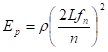, n=1, 2, 3…… (1)

where Ep is dynamic MOE of specimen, L is the length of the specimen, fn is the resonance frequency, and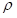is the density of the specimen.

The experiment was last carried out with the longitudinal transmission method as shown in Fig. 4. In the test, the sound transmission time propagating through the specimen was measured with a fast Fourier transform (FFT) analyzer. The sound velocity and dynamic MOE were calculated based on Eqs. 2 and 3 (Hu 2004),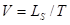(2)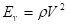(3)

where V is sound velocity, L is length of the specimen, T is transmission time, Ev is dynamic MOE, andis the density of the specimen.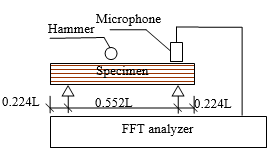Fig. 1. Out-plane flexural vibration test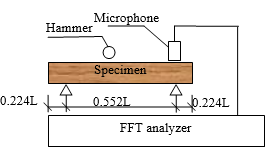Fig. 2. In-plane flexural vibration test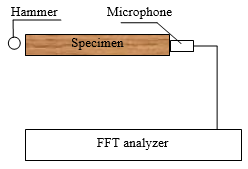Fig. 3. Longitudinal vibration test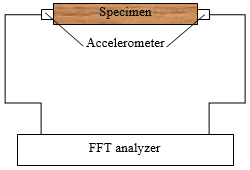Fig. 4. Longitudinal transmission test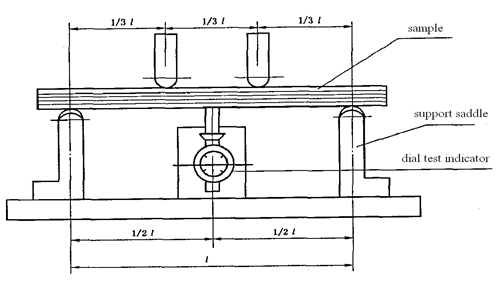Fig. 5. Vertical load static bending test

Static Bending Testing

The static bending tests were conducted on the LVL specimens in accordance with the Japanese Agricultural Standard (JAS: SE-11 No. 237: 2003) of Structural Laminated Veneer Lumber. The static modulus of rupture (MOR) in bending and modulus of elasticity (MOE) in bending were calculated based on Eq. 4 and 5,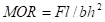(4)

where MOR is the modulus of rupture (MPa), is the maximum load (N), l is the span in bending between reaction points (mm), b is the cross-sectional width in the bending test (mm), and h is the cross-sectional thickness in the bending test (mm).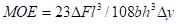(5)

In Eq. 5, MOE is the modulus of elasticity in bending perpendicular to the grain (GPa), ΔF is the increment of load on the regression line with a correlation coefficient of 0.99 or better (N), Δy is the increment of deformation corresponding to F2 – F(N), and lb, and h are the same as in Eq. 4.

Fuzzy Mathematical Analysis

It was necessary to strike each one of the LVL samples during the NDT testing because errors in veneer thickness in the assembly of LVL, varied distribution of early and late wood causing irregular compression, varying sizes of specimens, and the site of each strike not being at the same point on specimens would cause the NDT results to have fuzziness. However, these factors have been generally ignored when they were analyzed with classical mathematics.

In order to show the extent of the effects of these factors on NDT results, the NDT results were analyzed with fuzzy mathematics and compared with the results of classical mathematical analysis. Thus, the feasibility of using NDT to test the LVL mechanical properties was analyzed.

Fuzzy Regression and Nearness Analysis

Many factors need to be considered in engineering structural performance analysis. Any one factor can be regarded as the comprehensive analysis result of level down factors, thus a multi-level comprehensive evaluation can be obtained by this ranking method (Feng and Zeng 2008). The secondary fuzzy comprehensive evaluation model of NDT results is shown in Fig. 6. Its evaluation principle was obtained on the basis of the single stage fuzzy evaluation. In accordance with the single fuzzy comprehensive evaluation principle of fuzzy mathematics, the multistage fuzzy comprehensive evaluation steps for the evaluation object set E = {E1E2,…, En} included the following steps: establishing the factor set, establishing the weighted factor set, and establishing the membership matrix (for clarity, fuzzy set was abbreviated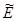, expressed as E).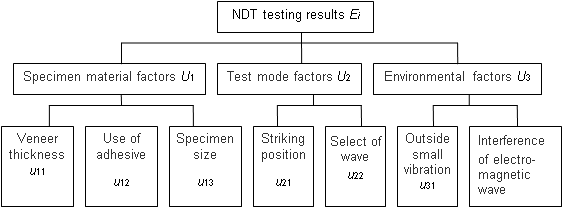Fig. 6. The secondary fuzzy comprehensive evaluation model of NDT testing results

Establishing the factor set

The factor set U, which influenced the NDT results of LVL, was divided into N classes according to different attributes, namely the primary factor set U = {U1U2, …, Um}. Each Uin the primary factor set contains ki different factors, i.e., the secondary factor set U= {ui1ui2, …, uik}, so there are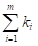factors in the factor set U.

Establishing the weighted factor set

The weighted factor set was confirmed according to the influence of each factor from low to high, namely the secondary factors weighted set a= {ai1ai2, …, aik}, = 1, 2,…, m. The membership rik = {rik1rik2, …, rikm} between uik and vwas obtained according to the evaluation set = {v1v2, …, vm} and formed a single factor evaluation matrix Ri, by the formula: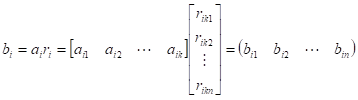(6)

Establishing the membership matrix

is the general object, whereas bik = {bik1bik2, …, bikn} values express the evaluation results of each factor of affiliated elements Ui, and serve as a member-ship matrix of the secondary fuzzy comprehensive evaluation. If R= b= {bi1bi2, …, bin}T(i = 1, 2, …, m), then = {R1R2, …, Rm} was the general membership matrix. Supposing that = {A1A2, …, Am} was the weight distribution set of each factor Ui in U, then the secondary fuzzy comprehensive evaluation results B can be obtained by the following formula.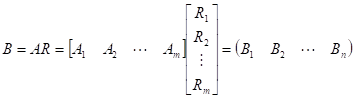(7)

Establishing the fuzzy regression equation

The general expression of a unary linear regression equation in classical mathematics is: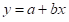(8)

Hypothetically, the results of the static bending test and an NDT method are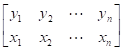(9)

When using the fuzzy regression analysis method, the best estimate is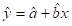(10)

where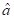and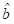are the best estimate of and b, respectively. For each group of NDT tests, the results were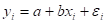（i = 1，2，…，n） (11)

where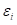is a regression equation error term and the error variable ε is a random fuzzy variable. To findand, hypothesis universe of discourse is as follows: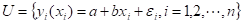(12)

Hypothesis R is a fuzzy subset in U, and clear estimation r of R can be found according to the sample value: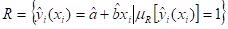(13)

The membership function of R is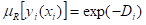(14)

where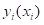is the test results of the sample value and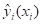is the unknown estimated value. The expression of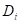is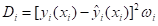(15)

where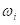is weight. Considering the data matching, take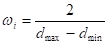(16)

where dmax and dmin are the maximum and minimum value of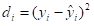(i = 1, 2, …, n). Therefore, when Di is greater, the weight of the corresponding NDT data is smaller when ascertaining the estimation of the linear regression equation. The best regression estimate is the target in this paper, i.e.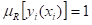, and then to try to obtain Di = 0, noted as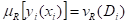(17)

The actual test result data attach to sample fuzzy subset R. According to the subjection degree maximum principle, objective function can be formed as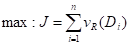(18)

Therefore,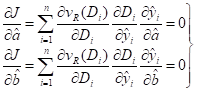(19)

Inserting Eq. 10 and 14-17 into Eq. 15 gives the following result: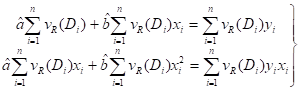(20)

Solving Eq. 20, one obtains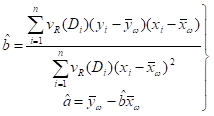(21)

where,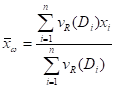，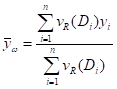Equation 21 is a system of implicit equations, andandcan be solved by a successive approximation method (Wang 2005).

Choice of solving method for fuzzy neartude

When X is a finite set, namely ={X1X2, …, Xm}, the common solving method of fuzzy neartude is (Peng and Sun 2002):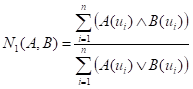(22)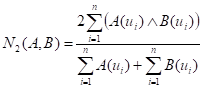(23)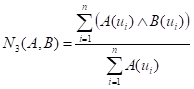(24)

RESULTS AND DISCUSSION

Static Analysis

Some past studies have shown that the mechanical properties of wood diminish with increases of moisture content (Dinwoodie et al. 1991; Mundy et al. 1998; Liu 2004). Therefore, the mechanical properties of LVL must be affected by the relative humidity of the environment. The effects of relative humidity on MOE and MOR of pine and poplar LVL with vertical load are shown in Fig. 7.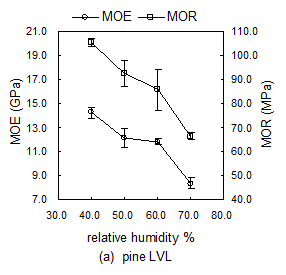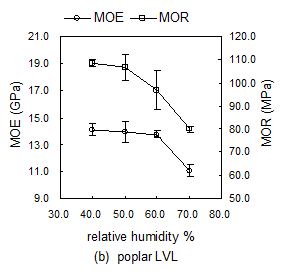Fig. 7. Effects of relative humidity on MOE and MOR of LVL with vertical load

According to Fig. 7, the MOE and MOR of pine and poplar LVL decreased with an increase of relative humidity. The largest decreasing amplitude of all MOE and MOR occurred from 60% to 70% relative humidity. The percent decrease was 22 to 42% from 40% to 70% relative humidity. In other words, there were relatively large effects of relative humidity on the mechanical properties of LVL.

Linear Regression Analysis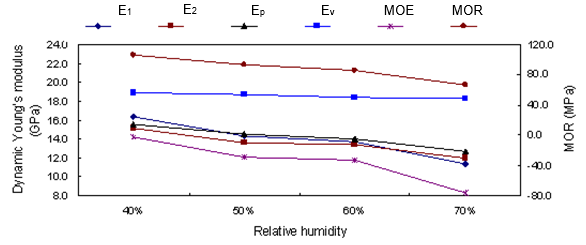Fig. 8. The average values of dynamic Young’s modulus and MOE with different relative humidities of pine LVL

Figure 8 describes the average values of Young’s modulus from five test results and MOR with different relative humidities. The values of the dynamic Young’s modulus obtained using NDT methods were larger than those of the static MOE obtained using the static bending test with the loading direction perpendicular to the glue-line. Eυ, the dynamic Young’s modulus obtained by the longitudinal transmission method, was the largest value. There was little difference between E1E2, and Ep, where E1 is the dynamic Young’s modulus from the out-plane flexural vibration method, E2 is the dynamic Young’s modulus from the in-plane flexural vibration method, and Ep is the dynamic Young’s modulus from the longitudinal vibration method. The trend curves of each NDT test result and MOE or MOR were very similar.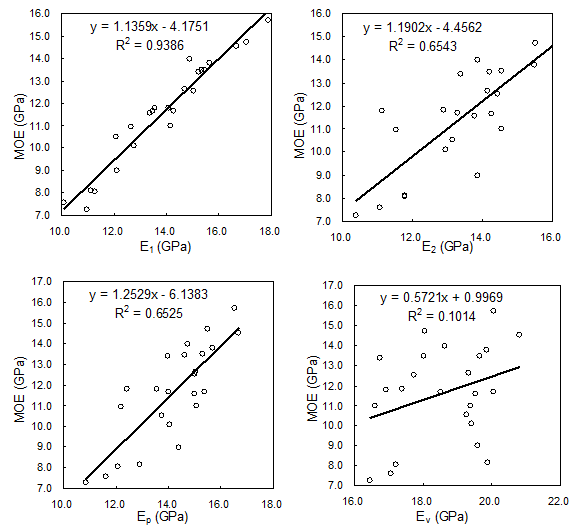Fig. 9. Regression curves between various dynamic Young’s modulus values and MOE of pine LVL

According to Fig. 8, the effects of the relative humidity on Young’s modulus and MOR of LVL were distinct. The five Young’s modulus values from the four test methods decreased with increasing relative humidity. When the relative humidity increased form 40% to 50% and 60% to 70%, most Young’s modulus values decreased greatly. When the relative humidity increased from 50% to 60%, the Young’s modulus values decreased inconspicuously. The regression curves between various dynamic Young’s modulus values and MOE of pine LVL are described by Fig. 9.

Due to the fuzziness of NDT results, each test result can be analyzed as a set using the weighted analysis of each factor. The classical regression analysis results were compared with large and small nearness analysis results in order to make the comparison between the fuzzy mathematical and the classical mathematical results more apparent. The obtained equations of linear regression, correlation coefficients, and fuzzy neartude between NDT results and MOE are shown in Tables 1 and 2.

Table 1. Linear Regression Formulas, Correlation Coefficients, and Fuzzy Neartude of Pine LVL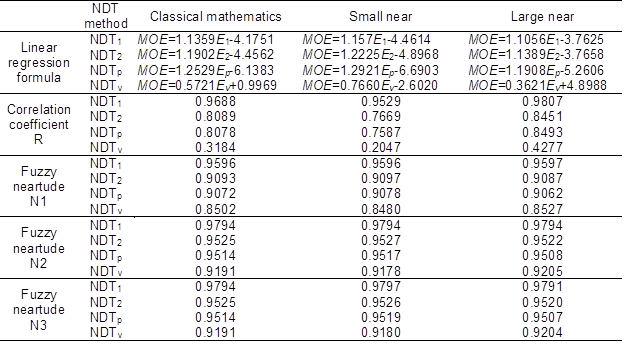where: NDT1 is out-plane flexural vibration method, NDT2 is in-plane flexural vibration method, NDTis longitudinal vibration method, and NDTv is longitudinal transmission method

According to Tables 1 and 2, the results from analysis of the correlation coefficient of classical mathematical linear regression were all between the large and small nearness analysis results. Therefore, the correlation coefficient between NDT results and static bending test results could be improved by using the large nearness fuzzy regression analysis. The correlation coefficient of the longitudinal transmission method on pine LVL was only 0.4277, still less than R22,0.01 = 0.515. The size relationship of each NDT method from fuzzy neartude analysis was the same as the size relationship of the correlation coefficient, namely NDT1 > NDT2 > NDTp > NDTv. Using fuzzy regression analysis had a certain effect on the correlation coefficient, but the effect on the fuzzy neartude was so small, almost a thousandth of a percentile, which can be regarded as no effect.

Table 2. Linear Regression Formulas, Correlation Coefficients, and Fuzzy Neartude of Poplar LVL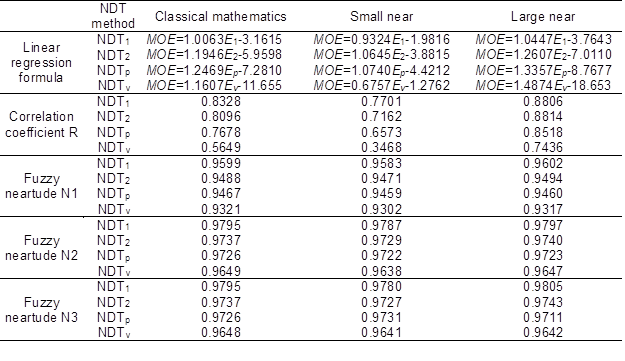Table 3 shows the equations for linear regression, all the correlation coefficients, and results for fuzzy neartude between NDT predictions and MOR values.

Table 3. The Linear Regression Formulas, Correlation Coefficients, and Fuzzy Neartude of Pine and Poplar LVL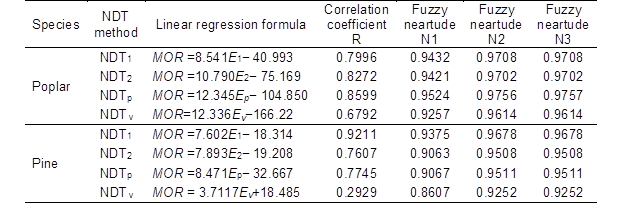As shown in Tables 1, 2, and 3, the size relationship of correlation coefficients and the fuzzy neartude of each of the NDT results on pine and poplar were the same, namely NDT1 > NDT2> NDTp > NDTv. The correlation coefficient between NDT results and MOE or MOR was more than R22,0.01 = 0.515, except for the longitudinal transmission on pine LVL. Therefore, there was a good linear correlation between NDT results and MOE or MOR of poplar and pine LVL.

Through the comparative analysis above, the conclusion can be drawn that the linear correlation coefficient can reflect the correlation of the two sets of data as a kind of test index of a linear regression analysis. To a certain extent, the fuzzy neartude can reflect the proximity between the static bending test results and the regression analysis results, but it cannot completely confirm the correlation between the two matching data sets. Therefore, the fuzzy neartude can be used as a reference for the feasibility analysis of linear regression results between the NDT results and MOE or MOR of pine and poplar LVL.

CONCLUSIONS

1. The linear correlation between various dynamic modulus results and MOE or MOR, except the longitudinal transmission on pine LVL, were better when the four NDT methods were used to test poplar and pine LVL mechanical properties in different relative humidity environments. The NDT methods can be used for MOE and MOR tests of poplar and pine LVL at different relative humidities.
2. The size relationship of each NDT method’s fuzzy neartude was the same as the size relationship of correlation coefficients. The fuzzy neartude can be used as a reference for the feasibility analysis of the linear regression between the NDT results and MOE or MOR of LVL.
3. The mechanical properties of poplar and pine LVL decreased when the relative humidity was increased. The most obvious decrease occurred from 60% to 70% relative humidity. The mechanical properties of poplar LVL was decreased by 21% to 37% and pine LVL was decreased by 25% to 41%, as relative humidity was increased from 40% to 70%. The effect of relative humidity on mechanical properties of pine LVL was greater than for poplar LVL.

ACKNOWLEDGMENTS

This project was supported by the National Natural Science Foundation of China (31170516, 31010103905), the Fundamental Research Funds for the Central Universities (DL12EB03), and the Scientific Research Fund of Heilongjiang Provincial Education Department (No: 12511488).

REFERENCES CITED

Bayraktar, E., Antolovich, S. D., and Bathias, C. (2008). “New developments in non-destructive controls of the composite materials and applications in manufacturing engineering” J. Mater. Process. Technol. 206(1-3), 30-44.

Cheng, F. C., and Hu, Y. C. (2011a). “Reliability analysis of timber structure design of poplar lumber with nondestructive testing methods,” BioResources 6(3), 3188-3198.

Cheng, F. C., and Hu, Y. C. (2011b). “Nondestructive test and prediction of MOE of FRP reinforced fast-growing poplar glulam,” Composites Science and Technology 71(8), 1163-1170.

Çolak, S., Çolakoĝlu, G, and Aydin, I. (2007). “Effects of logs steaming, veneer drying and aging on the mechanical properties of laminated veneer lumber (LVL),” Building and Environment 42(1), 93-98.

Dinwoodie, J. M., Robson, D. J., Paxton, B. H., and Higgins, J. S. (1991). “Creep in chipboard. Part 8: The effect of steady-state moisture content, temperature and level of stressing on the relative creep behaviour and creep modulus of a range of boards,” Wood Science and Technology 25(3), 225-238.

Dobmann, G., Meyendorf, N., and Schneider, E. (1997). “Nondestructive characterization of materials: A growing demand for describing damage and service-life-relevant aging process in plant components,” Nuclear Engineering and Design 171(1-3), 95-112.

Feng, Q. L., and Zeng, W. Y. (2008). “Fuzzy least absolute linear regression,” Journal of Beijing Normal University (Natural Science) 44(4), 348-353.

Hu, Y. C. (2004). Dynamic Properties and Nondestructive Test of Wood-based Composites, Northeast Forestry University Press, Harbin, China.

Hu, Y. C., Wang, F. H., Gu, J. Y., Liu, Y. X., and Nakao, T. (2005). “Nondestructive test and prediction of modulus of elasticity of veneer-overlaid particleboard composite,” Wood Sci. Technol. 39(6), 439-447.

Kurt, R., and Cil, M. (2012). “Effects of press pressure on glue line thickness and properties of laminated veneer lumber glued with melamine urea formaldehyde adhesive,” BioResources7(3), 4341-4349.

Li, B. H., and Zhang, X. J. (1992). “Fuzzy decision making analysis on the classification problems,” Fuzzy System and Mathematician 6(1), 81-86.

Liu, Y. X. (2004). Woodiness Resource Materials Science, China Forestry publishers, Beijing (in Chinese).

Mundy, J. S., Bonfield, P. W., Dinwoodie, J. M., and Paxton, B.H. (1998) “Modelling the creep behaviour of chipboard: The rheological approach,” Wood Science and Technology 32(4), 261-272.

Peng, Z. Z., and Sun, W. Y. (2002). Fuzzy Mathematics and Application, Wuhan University Press, Wuhan, China.

Ritschel, F., Brunner, A. J., and Niemz, P. (2013). “Nondestructive evaluation of damage accumulation in tensile test specimens made from solid wood and layered wood materials,” Composite Structures 95(1), 44-52.

Shukla, S. R., and Kamdem, D. P. (2008). “Properties of laminated veneer lumber (LVL) made with low density hardwood species: Effect of the pressure duration,” Holz Roh Werkst.66(2), 119-127.

Shukla, S. R., and Kamdem, D. P. (2009). “Properties of laboratory made yellow poplar (Liriodendron tulipifera) laminated veneer lumber. Effect of the adhesives,” Eur. J. Wood Prod.67(4), 397-405.

Souza, F. D., Menezzi, C. H. S. D., and Júnior, G. B. (2011). “Material properties and nondestructive evaluation of laminated veneer lumber (LVL) made from Pinus oocarpa and P. kesiya,” Eur. J. Wood Prod. 69(2), 183-192.

Wang, C. (2007). “Requirements for the accreditation of a nondestructive testing laboratory and the control of testing process,” Nondestructive Testing (Chinese) 29(7), 423-426.

Wang, P., Li, A. G., Cai, M. F., and Yang, T. (2005). “Random-fuzzy monovariant linear regression method for determining the shear strength parameters of rock,” Chinese Journal of Rock Mechanics and Engineering 24(4), 547-552.

Yin, Y. F., Nagao, H., Liu, X. L., and Nakai, T. (2010). “Mechanical properties assessment of Cunninghamia lanceolata plantation wood with three acoustic-based nondestructive methods,” Journal of Wood Science 56(1), 33-40.

Article submitted: February 22, 2013; Peer review completed: April 9, 2013; Revised version received and accepted: April 16, 2013; Published: April 23, 2013.